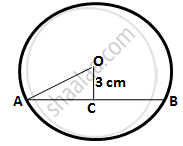Share

# A Chord of Length 8 Cm is Drawn at a Distance of 3 Cm from the Centre of a Circle. Calculate the Radius of the Circle. - Mathematics

Course

#### Question

A chord of length 8 cm is drawn at a distance of 3 cm from the centre of a circle. Calculate the radius of the circle.

#### Solution

Let AB be the chord and O be the centre of the circle.
Let OC be the perpendicular drawn from O to AB.We know, that the perpendicular to a chord, from the centre of a circle, bisects the chord.
∴ AB = 8 cm

⟹ AC = CB =("AB")/2

⟹ AC = CB =8/2

⟹ AC = CB = 4 cm

In ∆OCA,

OA2 = OC2 + AC(By Pythagoras theorem)

⟹ OA2 = (4)2 + (3)2 = 25

⟹ OA = 5 cm

Hence, radius of the circle is 5 cm.

Is there an error in this question or solution?